## Abstract

Numerical computation of unsteady laminar three-dimensional natural convection and entropy generation in an inclined cubical trapezoidal air-filled cavity is performed for the first time in this work. The vertical right and left sidewalls of the cavity are maintained at constant cold temperatures. The lower wall is subjected to a constant hot temperature, while the upper one is considered insulated. Computations are performed for Rayleigh numbers varied as 103 ⩽ Ra ⩽ 105, while the trapezoidal cavity inclination angle is varied as 0° ⩽ Φ ⩽ 180°. Prandtl number is considered constant at Pr = 0.71. Second law of thermodynamics is applied to obtain thermodynamic losses inside the cavity due to both heat transfer and fluid friction irreversibilities. The variation of local and average Nusselt numbers is presented and discussed, while, streamlines, isotherms and entropy contours are presented in both two and three-dimensional pattern. The results show that when the Rayleigh number increases, the flow patterns are changed especially in three-dimensional results and the flow circulation increases. Also, the inclination angle effect on the total entropy generation becomes insignificant when the Rayleigh number is low. Moreover, when the Rayleigh number increases the average Nusselt number increases.

## Keywords

Transient natural convection; Trapezoidal cavity; Three-dimensional flow; Entropy generation; Second law analysis

## Nomenclature

Symbol- Description (unit)

g- gravitational acceleration (m/s2)

H- height of the trapezoidal cubical cavity (m)

k- fluid thermal conductivity (W/m °C)

n- unit vector normal to the wall

L- length of the cubical trapezoidal cavity (m)

Nu- Nusselt number

${\textstyle N_{s}}$- dimensionless local generated entropy

Pr- Prandtl number

${\textstyle {\overset {\rightarrow }{q}}}$- heat flux (W/m2)

Ra- Rayleigh number

S- dimensionless entropy generation

${\textstyle S_{\mbox{gen}}^{'}}$- generated entropy (Kj/kg K)

T- dimensionless temperature ${\textstyle \left[(T^{'}-T_{c}^{'})/(T_{h}^{'}-T_{c}^{'})\right]}$

To- bulk temperature ${\textstyle \left[T_{o}=(T_{c}^{'}+T_{h}^{'})/2\right]}$

t- dimensionless time (${\textstyle t^{'}\cdot \alpha /L^{2}}$)

${\textstyle {\overset {\rightarrow }{V}}}$- dimensionless velocity vector ${\textstyle \left({\overset {\rightarrow }{V}}^{'}\cdot L/\alpha \right)}$

x- dimensionless Cartesian coordinate in x  -direction (${\textstyle x^{'}/L}$)

y- dimensionless Cartesian coordinate in y  -direction (${\textstyle y^{'}/L}$)

z- dimensionless Cartesian coordinate in z  -direction (${\textstyle z^{'}/L}$)

### Greek symbols

α- thermal diffusivity (m2/s)

β- thermal expansion coefficient (K−1)

${\textstyle \Delta T}$- dimensionless temperature difference

${\textstyle {\phi }^{'}}$- dissipation function

${\textstyle \Phi }$- trapezoidal cavity inclination angle (degree)

υ- fluid kinematic viscosity (m2/s)

${\textstyle {\overset {\rightarrow }{\psi }}}$- dimensionless vector potential (${\textstyle {\overset {\rightarrow }{\psi }}^{'}/\alpha }$)

${\textstyle \mu }$- dynamic viscosity (kg/m s)

${\textstyle {\overset {\rightarrow }{\omega }}}$- dimensionless vorticity (${\textstyle {\overset {\rightarrow }{\omega }}^{'}\cdot \alpha /L^{2}}$)

### Subscripts

av- average

c- cold

fr- friction

h- hot

Loc- local

th- thermal

tot- total

x, y, z- Cartesian coordinates

### Superscripts

${\textstyle {'}}$- dimensional variable

## 2. Mathematical model

### 2.1. Definition of geometrical configuration

The three-dimensional natural convection and entropy generation problem inside an inclined cubical trapezoidal cavity of height (H) and length (L) filled with air [Pr = 0.71] are investigated in the present work as shown in Fig. 1. The vertical right and left sidewalls of a trapezoidal cavity are kept at isothermal cold temperatures (Tc). The upper and lower walls are considered insulated and subjected to an isothermal hot temperature (Th) respectively. The flow field inside the cubical cavity is considered three-dimensional, Newtonian, unsteady, incompressible and laminar. From the other side, the heat transfer due to thermal gradients and friction effects causes that the cubical trapezoidal cavity is subjected to a loss in energy and as a result an entropy generation is induced. The Rayleigh number is varied as 103 ⩽ Ra ⩽ 105, while the trapezoidal cavity inclination angle is varied as 0° ⩽ Φ ⩽ 180°. The Rayleigh number relates the relative magnitude of viscous and buoyancy forces in the fluid. The natural convection occurs when buoyancy forces are greater than viscous forces. The fluid inside the cubical cavity is assumed to have constant thermo-physical properties and Boussinesq approximation is used to model the density variation.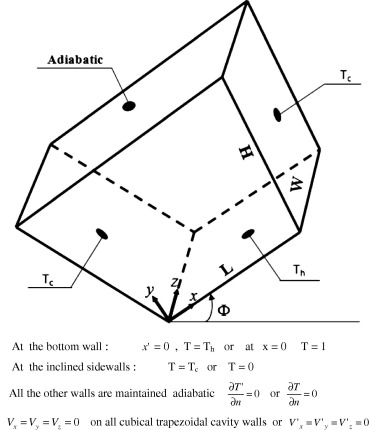Figure 1. Schematic diagram of the present problem.

### 2.2. Mathematical model and the numerical solution

To start the numerical approach, the vorticity-vector potential formalism ${\textstyle \left({\overset {\rightarrow }{\psi }}-{\overset {\rightarrow }{\omega }}\right)}$ is considered in the present work which allows in a three-dimensional geometry together with the elimination of the pressure term. Vector potential and the vorticity are defined respectively by the following two equations:

 ${\overset {\rightarrow }{\omega }}^{'}={\overset {\rightarrow }{\nabla }}\times {\overset {\rightarrow }{V}}^{'}\quad \quad {\mbox{and}}\quad \quad {\overset {\rightarrow }{V}}^{'}=$${\overset {\rightarrow }{\nabla }}\times {\overset {\rightarrow }{\psi }}^{'}$
(1)

The construction of these equations is described in more details in Ghachem et al. . The dimensionless governing equations can be written as follows:

 $-{\overset {\rightarrow }{\omega }}={\nabla }^{2}{\overset {\rightarrow }{\psi }}$
(2)

 ${\frac {\partial {\overset {\rightarrow }{\omega }}}{\partial t}}+$$({\overset {\rightarrow }{V}}\cdot \nabla ){\overset {\rightarrow }{\omega }}-$$({\overset {\rightarrow }{\omega }}\cdot \nabla ){\overset {\rightarrow }{V}}=$$\Delta {\overset {\rightarrow }{\omega }}+{\mbox{Ra}}\cdot Pr\cdot \left[{\begin{array}{l}{\frac {\partial T}{\partial z}}cos\Phi \\-{\frac {\partial T}{\partial z}}sin\Phi \\-{\frac {\partial T}{\partial x}}cos\Phi +{\frac {\partial T}{\partial y}}sin\Phi \end{array}}\right]$
(3)

 ${\frac {\partial T}{\partial t}}+{\overset {\rightarrow }{V}}\cdot \nabla T=$${\nabla }^{2}T$
(4)

where

 $Pr={\frac {\nu }{\alpha }}\quad {\mbox{and}}\quad {\mbox{Ra}}=$${\frac {g\beta (T_{h}-T_{C})L^{3}}{\nu \cdot \alpha }}$

Moreover, the dimensionless temperature, dimensionless time, dimensionless velocity, dimensionless vector potential and dimensionless vorticity are defined respectively as

 $T={\frac {\left(T^{'}-T_{c}^{'}\right)}{\left(T_{h}^{'}-T_{c}^{'}\right)}}\quad t=$${\frac {t^{'}\cdot \alpha }{L^{2}}}\quad {\overset {\rightarrow }{V}}=$${\frac {{\overset {\rightarrow }{V}}^{'}\cdot L}{\alpha }}\quad {\overset {\rightarrow }{\psi }}=$${\frac {{\overset {\rightarrow }{\psi }}^{'}}{\alpha }}\quad {\overset {\rightarrow }{\omega }}=$${\frac {{\overset {\rightarrow }{\omega }}^{'}\cdot \alpha }{L^{2}}}$

while, the dimensionless Cartesian coordinates in x, y and z directions are defined as

 $x={\frac {x^{'}}{L}}\quad y={\frac {y^{'}}{L}}\quad z=$${\frac {z^{'}}{L}}$

#### 2.2.1. Boundary conditions

The boundary conditions for the present problem are given as follows:

Temperature:

 $T=1\quad {\mbox{at}}\quad x=0\quad [{\mbox{at bottom wall}}]{\mbox{,}}\quad T=$$0\quad {\mbox{at the inclined sidewalls}}$
 ${\frac {\partial T}{\partial n}}=0\quad {\mbox{on all other walls}}\quad ({\mbox{adiabatic}})$

Vorticity:

 ${\omega }_{x}=0{\mbox{,}}\quad {\omega }_{y}=-{\frac {\partial V_{z}}{\partial x}}{\mbox{,}}\quad {\omega }_{z}=$${\frac {\partial V_{y}}{\partial x}}\quad {\mbox{at}}\quad x=$$0\quad {\mbox{and}}\quad 1$
 ${\omega }_{x}={\frac {\partial V_{z}}{\partial y}}{\mbox{,}}\quad {\omega }_{y}=$$0{\mbox{,}}\quad {\omega }_{z}=-{\frac {\partial V_{x}}{\partial y}}\quad {\mbox{at inclined sidewalls}}$
 ${\omega }_{x}=-{\frac {\partial V_{y}}{\partial z}}{\mbox{,}}\quad {\omega }_{y}=$${\frac {\partial V_{x}}{\partial z}}{\mbox{,}}\quad {\omega }_{z}=$$0\quad {\mbox{at}}\quad z=0\quad {\mbox{and}}\quad 1$

Vector potential:

 ${\frac {\partial {\Psi }_{x}}{\partial x}}={\Psi }_{y}={\Psi }_{z}=$$0\quad {\mbox{at}}\quad x=0\quad {\mbox{and}}\quad 1$
 ${\Psi }_{x}={\frac {\partial {\Psi }_{y}}{\partial y}}={\Psi }_{z}=$$0\quad {\mbox{at inclined sidewalls}}$
 ${\Psi }_{x}={\Psi }_{y}={\frac {\partial {\Psi }_{z}}{\partial z}}=$$0\quad {\mbox{at}}\quad z=0\quad {\mbox{and}}\quad 1$

Velocity:

 $V_{x}=V_{y}=V_{z}=0\quad {\mbox{on all cubical trapezoidal cavity walls}}$

The generated entropy (${\textstyle S_{\mbox{gen}}^{'}}$) is written by Kolsi et al.  in the following form:

 $S_{\mbox{gen}}^{'}=-{\frac {1}{T^{{'}2}}}\cdot {\overset {\rightarrow }{q}}\cdot {\overset {\rightarrow }{\nabla }}T^{'}+$${\frac {\mu }{T^{'}}}\cdot {\phi }^{'}$
(5)

The first term represents the generated entropy due to the temperature gradient, while the second one is due to the friction effects. The heat flux vector is given by

 ${\overset {\rightarrow }{q}}=-k\cdot gra{\overset {\rightarrow }{d}}T$
(6)

The dissipation function (${\textstyle {\phi }^{'}}$) is written as follows:

 ${\phi }^{'}=2\left[{\left({\frac {\partial V_{x}^{'}}{\partial x^{'}}}\right)}^{2}+\right.$$\left.{\left({\frac {\partial V_{y}^{'}}{\partial y^{'}}}\right)}^{2}+\right.$$\left.{\left({\frac {\partial V_{z}^{'}}{\partial z^{'}}}\right)}^{2}\right]+$${\left({\frac {\partial V_{y}^{'}}{\partial x^{'}}}+{\frac {\partial V_{x}^{'}}{\partial y^{'}}}\right)}^{2}+$${\left({\frac {\partial V_{z}^{'}}{\partial y^{'}}}+{\frac {\partial V_{y}^{'}}{\partial z^{'}}}\right)}^{2}+$${\left({\frac {\partial V_{x}^{'}}{\partial z^{'}}}+{\frac {\partial V_{z}^{'}}{\partial x^{'}}}\right)}^{2}$
(7)

Therefore, the generated entropy ${\textstyle \left(S_{\mbox{gen}}^{'}\right)}$ is written as

 $S_{\mbox{gen}}^{'}={\frac {k}{T_{0}^{2}}}\left[{\left({\frac {\partial T^{'}}{\partial x^{'}}}\right)}^{2}+\right.$$\left.{\left({\frac {\partial T^{'}}{\partial y^{'}}}\right)}^{2}+\right.$$\left.{\left({\frac {\partial T^{'}}{\partial z^{'}}}\right)}^{2}\right]+$${\frac {\mu }{T_{0}}}\left\{2\left[{\left({\frac {\partial V_{x}^{'}}{\partial x^{'}}}\right)}^{2}+\right.\right.$$\left.\left.{\left({\frac {\partial V_{y}^{'}}{\partial y^{'}}}\right)}^{2}+\right.\right.$$\left.\left.{\left({\frac {\partial V_{z}^{'}}{\partial z^{'}}}\right)}^{2}\right]+\right.$$\left.{\left({\frac {\partial V_{y}^{'}}{\partial x^{'}}}+{\frac {\partial V_{x}^{'}}{\partial y^{'}}}\right)}^{2}+\right.$$\left.{\left({\frac {\partial V_{z}^{'}}{\partial y^{'}}}+{\frac {\partial V_{y}^{'}}{\partial z^{'}}}\right)}^{2}+\right.$$\left.{\left({\frac {\partial V_{x}^{'}}{\partial z^{'}}}+{\frac {\partial V_{z}^{'}}{\partial x^{'}}}\right)}^{2}\right\}$
(8)

The dimensionless local generated entropy (${\textstyle N_{s}}$) is written as follows:

 $N_{s}=S_{\mbox{gen}}^{'}{\frac {1}{k}}{\left({\frac {{LT}_{0}}{\Delta T}}\right)}^{2}$
(9)

where

 $N_{s}=\left[{\left({\frac {\partial T}{\partial x}}\right)}^{2}+\right.$$\left.{\left({\frac {\partial T}{\partial y}}\right)}^{2}+\right.$$\left.{\left({\frac {\partial T}{\partial z}}\right)}^{2}\right]+$$\varphi \cdot \left\{2\left[{\left({\frac {\partial V_{x}}{\partial x}}\right)}^{2}+\right.\right.$$\left.\left.{\left({\frac {\partial V_{y}}{\partial y}}\right)}^{2}+\right.\right.$$\left.\left.{\left({\frac {\partial V_{z}}{\partial z}}\right)}^{2}\right]+\right.$$\left.\left[{\left({\frac {\partial V_{y}}{\partial x}}+{\frac {\partial V_{x}}{\partial y}}\right)}^{2}+\right.\right.$$\left.\left.{\left({\frac {\partial V_{z}}{\partial y}}+{\frac {\partial V_{y}}{\partial z}}\right)}^{2}+\right.\right.$$\left.\left.{\left({\frac {\partial V_{x}}{\partial z}}+{\frac {\partial V_{z}}{\partial x}}\right)}^{2}\right]\right\}$
(10)

where ${\textstyle \varphi ={\frac {\mu {\alpha }^{2}T_{0}}{L^{2}k\Delta T^{2}}}}$ is the irreversibility coefficient.

The first term of (Ns) represents the local irreversibility due to the temperature gradients, and it is noted as (Ns–th). The second term of (Ns) represents the contribution of the viscous effects in the irreversibility and it is noted as (Ns–fr). It is useful to mention that Eq. (10) gives a good idea on the profile and the distribution of the dimensionless local generated entropy (Ns  ). Also, the total dimensionless generated entropy (${\textstyle S_{\mbox{tot}}}$) is written as

 $S_{\mbox{tot}}={\int }_{v}N_{s}dv={\int }_{v}\left(N_{s-{\mbox{th}}}+\right.$$\left.N_{s-{\mbox{fr}}}\right)dv=S_{\mbox{th}}+S_{\mbox{fr}}$
(11)

The local and average Nusselt numbers are given by

 ${\mbox{Nu}}={\frac {\partial T}{\partial y}}_{y=0}\quad {\mbox{and}}\quad {\mbox{Nu}}_{\mbox{av}}=$${\frac {L}{W}}{\int }_{0}^{1}{\int }_{0}^{W/L}{\mbox{Nu}}\cdot dx\cdot dz$
(12)

The mathematical model described above was written by a FORTRAN program. The control volume finite difference method is used to discretize governing (2), (3), (4) and (10) respectively. The central-difference scheme is used for treating convective terms while the fully implicit procedure is used to discretize the temporal derivatives. The grids are considered uniform in all directions with clustering nodes on boundaries. The successive relaxation iteration scheme is used to solve the resulting non-linear algebraic equations. The time step (10−4) and spatial mesh (71 × 71 × 71) are utilized to carry out all the numerical tests. The solution is considered acceptable when the following convergence criterion is satisfied for each step of time:

 $\sum _{i}^{1{\mbox{,}}2{\mbox{,}}3}{\frac {max\left|{\psi }_{i}^{n}-{\psi }_{i}^{n-1}\right|}{max\left|{\psi }_{i}^{n}\right|}}+$$max\left|T_{i}^{n}-T_{i}^{n-1}\right|\leq {10}^{-4}$
(13)

In order to check the accuracy of our code, a verification is performed by using the present numerical algorithm to simulate the same problem considered by Basak et al.  using the same geometry and boundary conditions for laminar natural convection flow in a two-dimensional trapezoidal porous enclosure. The comparison for both streamlines and isotherms is shown in Fig. 2 using the dimensionless parameters: Pr = 0.7, Da = 10−3, Ra = 105 and ${\textstyle \Phi =0{\mbox{°}}}$ and a good agreement is obtained. Therefore, the computational procedure is ready and can predict the three-dimensional natural convection and the entropy generation in an inclined trapezoidal cavity and as a result, the previous verification gives a good interest in the present numerical model to deal with the recent physical problem.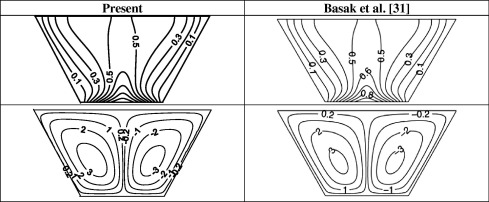Figure 2. Comparison of streamlines and isotherms at Pr = 0.7, Da = 10−3, Ra = 105 and ${\textstyle \Phi =0{\mbox{°}}}$.

## 3. Results and discussion

The unsteady laminar three-dimensional natural convection and entropy generation in an inclined cubical trapezoidal air-filled cavity are investigated numerically in this work. The effect of Rayleigh number (Ra) and cavity inclination angle (${\textstyle \Phi }$) on the fluid flow, heat transfer and entropy generation has been performed. The results were presented via streamlines, trajectory of particles, isotherms, entropy contours together with local and average Nusselt numbers.

### 3.1. Effect of Rayleigh number on the thermal field

Fig. 3 illustrates isosurfaces of temperature around the all geometry (left) and isotherms along the mid-section [Z = 0.5] (right) in the cubical trapezoidal cavity [${\textstyle \Phi =0{\mbox{°}}}$] for various values of Rayleigh number [(a) Ra = 103, (b) Ra = 104 and (c) Ra = 105]. When the Rayleigh number is low [Ra = 103] or when the effect of natural convection is slight, the isotherms are in general smooth, straight lines and parallel to cavity sidewalls. This behavior is due to flow weakness when the Rayleigh number is low [Ra = 103]. It can be seen from Fig. 3, that isotherms emanate from the lower wall (or base) where the heat source exists and end on cold right and left sidewalls, indicating the heat flow path. Heat conduction is the dominant mechanism of heat transfer inside the cubical cavity in this case. But, as the Rayleigh number increases to [Ra = 104 and Ra = 105], buoyancy force dominates over viscous force leading to increase the natural convection effect. Therefore, the shape of isotherms begins to deviate sharply from uniform one encountered in the case where [Ra = 103]. This is due to the fact that strong circulation occurs when the Rayleigh number is high. The concentration of isotherms adjacent to the lower wall increases as the Rayleigh number increases illustrating high amount of heat and large temperature gradient adjacent to the lower wall of the cubical trapezoidal cavity. Therefore, a thermal boundary layer is constructed at this region and can be observed especially when [Ra = 105]. Heat convection is the dominant mechanism of heat transfer in this case. Therefore, it can be concluded that there is a clear conversion in isotherms pattern from uniform smooth shape to a high confuse one as the Rayleigh number increases. This behavior gives a clear approval of natural convection effect on the thermal field inside the cubical trapezoidal cavity.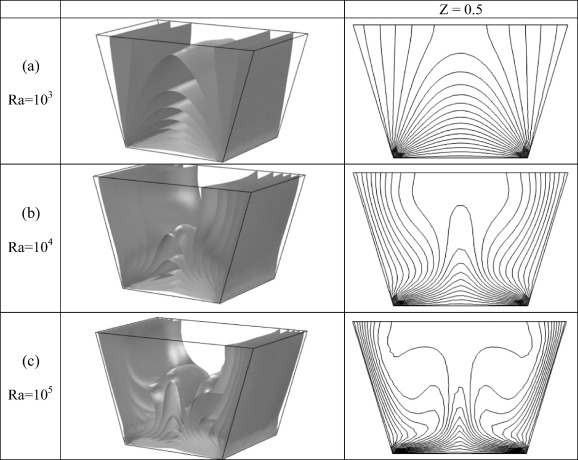Figure 3. The isosurfaces of temperature around the all geometry (left) and isotherms along the mid-section (right) in the cubical trapezoidal cavity [${\textstyle \Phi =0{\mbox{°}}}$] for various values of Rayleigh number (a) Ra = 103, (b) Ra = 104 and (c) Ra = 105.

### 3.2. Effect of Rayleigh number on the flow field

Fig. 4 presents the trajectory of particles around the all geometry (left) and streamlines along the mid section [Z = 0.5] (right) in the cubical trapezoidal cavity [${\textstyle \Phi =0{\mbox{°}}}$] for various values of Rayleigh number [(a) Ra = 103, (b) Ra = 104 and (c) Ra = 105]. According to the natural convection effect, the flow field begins to move from the hot lower wall until it arrives to the insulated upper wall. Then, it changes its direction and moves toward the cold right and left sidewalls after passing secondly adjacent to the hot lower wall. This cyclic motion of the flow field generates the re-circulating vortices which occupy all span of the cubical trapezoidal cavity as seen in Fig. 4. It can be noticed that the air stream which moves upward adjacent to the hot lower wall separates into two re-circulating vortices as indicated in 3D results. This flow behavior can be seen for all the considered values of Rayleigh number, but there is a difference in the shape of vortices related with the value of Rayleigh number. In the case of low Rayleigh number [Ra = 103], the effect of buoyancy force [which is generated due to the temperature difference] is slight and the flow circulation is uniform. Therefore, the convection effect is weak. Now, when the Rayleigh number increases to [Ra = 104 and Ra = 105] or when the effect of buoyancy force is significant, the frictional resistance to the fluid motion diminishes gradually. Therefore, the flow circulation becomes less uniform. This notation is in a good agreement with the results of Fusegi et al. . It can be observed that, the strict uniform pattern of re-circulating vortices encountered at [Ra = 103] is broken due to the strong effect of natural convection inside the cubical trapezoidal cavity, while, a weak fluid motion is noticed at the edges of the cubical cavity for all the considered range of Rayleigh number. Moreover, it can be seen also that the difference between the two-dimensional and three-dimensional results becomes clear as Rayleigh number increases from [Ra = 103] to [Ra = 105].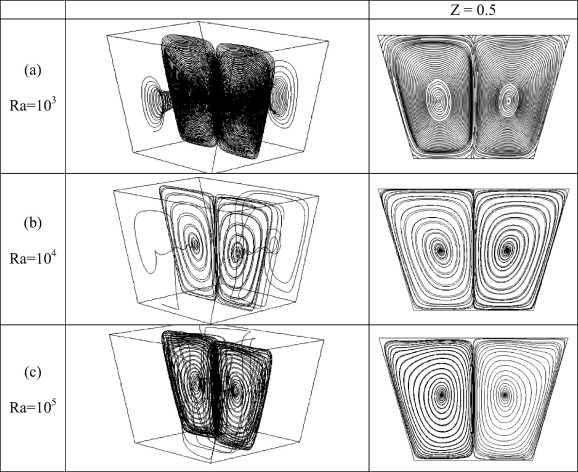Figure 4. The trajectory of particles around the all geometry (left) and streamlines along the mid-section (right) in the cubical trapezoidal cavity [${\textstyle \Phi =0{\mbox{°}}}$] for various values of Rayleigh number (a) Ra = 103, (b) Ra = 104 and (c) Ra = 105.

### 3.3. Effect of Rayleigh number on local and average Nusselt numbers

Fig. 5 demonstrates the profiles of the local Nusselt numbers (NuLoc) at the hot lower wall in the cubical trapezoidal cavity [${\textstyle \Phi =0{\mbox{°}}}$] for various values of Rayleigh number [(a) Ra = 103, (b) Ra = 104 and (c) Ra = 105]. These profiles reveal that the local Nusselt number is linear at low Rayleigh number [Ra = 103] .This indicates that the heat is transferred inside the cubical trapezoidal cavity by pure conduction. But, as the Rayleigh number increases to [Ra = 104 and Ra = 105], local Nusselt number profiles start to change its shape indicating the onset of natural convection. However, at [Ra = 104], the local Nusselt profiles are still in the transition process from conduction mode to a fully convection one. Moreover, it is interesting to observe high values of the local Nusselt number adjacent to the cavity hot lower wall due to the existence of heat source at this place. Fig. 6 illustrates the effect of Rayleigh number on the average Nusselt number (Nuav) in the cubical trapezoidal cavity at [${\textstyle \Phi =0{\mbox{°}}}$]. The average Nusselt number is a measure of the heat transfer rate inside the cubical trapezoidal cavity. It can be seen as expected, that the average Nusselt number increases as the Rayleigh number increases. This is because the natural convection and flow circulation enhance when the Rayleigh number increases. Therefore, the highest average Nusselt number corresponds to the highest Rayleigh number and vice versa. The reason of this behavior is due to the increase in the temperature gradient when the Rayleigh number increases. This increase leads to the average Nusselt number increasing.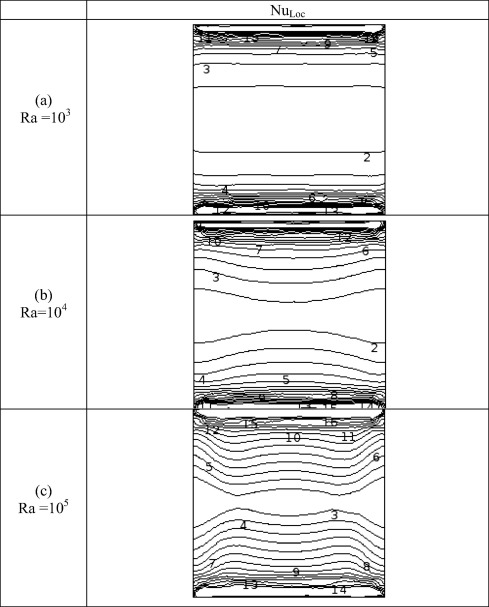Figure 5. The variation in the local Nusselt numbers at the hot lower wall in the cubical trapezoidal cavity [${\textstyle \Phi =0{\mbox{°}}}$] for various values of Rayleigh number [(a) Ra = 103, (b) Ra = 104 and (c) Ra = 105].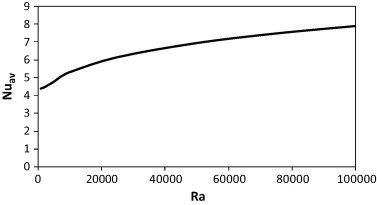Figure 6. The variation in the average Nusselt numbers in the cubical trapezoidal cavity [${\textstyle \Phi =0{\mbox{°}}}$] for various values of Rayleigh number.

### 3.4. Effect of Rayleigh number on entropy generation

Fig. 7 displays iso-surfaces (left) and isolines (right) of the entropy generation due to the heat transfer (Sth) in the cubical trapezoidal cavity [${\textstyle \Phi =0{\mbox{°}}}$] for various values of Rayleigh number [(a) Ra = 103, (b) Ra = 104 and (c) Ra = 105]. It can be noticed that the entropy generation contours extend deeply inside the cubical trapezoidal cavity as the Rayleigh number increases. This is due to the increase in the heat transfer losses with increasing the Rayleigh number, which leads to increase the entropy generation. Moreover, it can be seen that the entropy generation contours increase near the lower wall due to the heat source existence, while, they weak adjacent to the left and right cavity sidewalls even at [Ra = 105]. Furthermore, it can be seen from Fig. 7, that there is a space region in the cubical cavity core. This is because of stagnant or low fluid velocity. Fig. 8 demonstrates iso-surfaces (left) and isolines (right) of the entropy generation due to the friction (Sfr) in the cubical trapezoidal cavity [${\textstyle \Phi =0{\mbox{°}}}$] for various values of Rayleigh number [(a) Ra = 103, (b) Ra = 104 and (c) Ra = 105]. It can be seen that the entropy generation contours due to the fluid friction are concentrated strongly adjacent to the cavity right and left sidewalls. This is due to the existence of boundary layer which leads to increase the entropy generation due to the friction there. This observation is in a good agreement with the results of Mukhopadhyay . It is interesting to see that, the total entropy generation (Stot) and the entropy generation due to heat transfer (Sth) have an approximately similar pattern when [Ra = 103 and Ra = 104]. This behavior indicates the domination of the irreversibility due to the heat transfer at this range of Rayleigh number. But, when the Rayleigh number is high [Ra = 105] the total entropy generation (Stot) has a similar pattern with the entropy generation due to the fluid friction (Sfr). This behavior indicates the domination of the irreversibility due to the fluid friction at high Rayleigh number. This behavior will be highlighted in more detail later. Fig. 9 shows iso-surfaces (left) and isolines (right) of the total entropy generation (Stot) in the cubical trapezoidal cavity [${\textstyle \Phi =0{\mbox{°}}}$] for various values of Rayleigh number [(a) Ra = 103, (b) Ra = 104 and (c) Ra = 105]. From this figure, one can notice that when the Rayleigh number is low [Ra = 103], the entropy contours are concentrated near the lower wall in a similar behavior to their corresponding contours noticed in Fig. 7. The main conclusion of this behavior is that the entropy generation due to heat transfer (Sth) is high and dominant, while the entropy generation due to the fluid friction (Sfr) is weak. Therefore, the contribution of the entropy generation due to heat transfer (Sth) is greater than the entropy generation due to the fluid friction (Sfr) which makes the contours of the total entropy generation (Stot) similar to the contours of entropy generation due to heat transfer (Sth). At [Ra = 104], the total entropy generation contours (Stot) are somewhat similar to the corresponding contours which are seen in Figure 7 and Figure 8 respectively. So, in this case the contribution of entropy generations due to heat transfer and fluid friction is comparable. But when the Rayleigh number is high [Ra = 105], the entropy contours are concentrated adjacent to the cavity right and left sidewalls in a similar behavior to their corresponding contours noticed in Fig. 8. The main conclusion of this behavior is that the entropy generation due to the fluid friction (Sfr) is high and dominant, while the entropy generation due to the heat transfer (Sth) is weak. Therefore, the contribution of the entropy generation due to fluid friction (Sfr) is greater than the entropy generation due to the heat transfer (Sth) which makes the contours of the total entropy generation (Stot) similar to the contours of the entropy generation due to fluid friction (Sfr). Fig. 10 presents the variation of different entropy generation [Sth, Sfr and Stot] in the cubical trapezoidal cavity with various values of Rayleigh number. It can be seen that the entropy generation rate due to the heat transfer (Sth) increases slightly as the Rayleigh number increases. From the other side, there is a clear increase of entropy generation due to friction (Sfr) and the total entropy generation (Stot) when the Rayleigh number increases. This is due to the slight increase in the heat transfer losses and high increase in fluid friction losses with increasing the Rayleigh number. Therefore, the maximum value of the total entropy generation (Stot) and entropy generation due to friction (Sfr) occurs at maximum value of Rayleigh number [i.e., Ra = 105].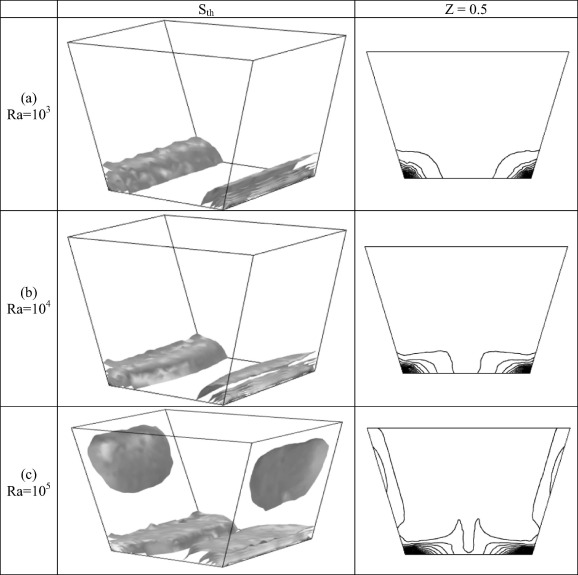Figure 7. The iso-surfaces (left) and isolines (right) of the entropy generation due to the heat transfer (Sth) in the cubical trapezoidal cavity [${\textstyle \Phi =0{\mbox{°}}}$] for various values of Rayleigh number [(a) Ra = 103, (b) Ra = 104 and (c) Ra = 105].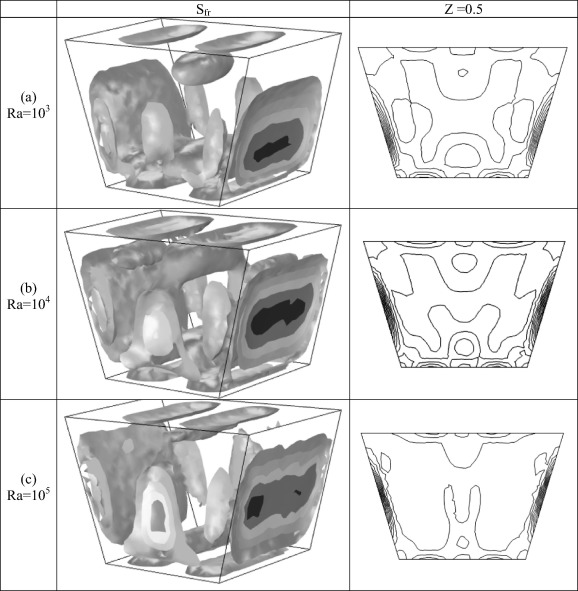Figure 8. The iso-surfaces (left) and isolines (right) of the entropy generation due to the friction (Sfr) in the cubical trapezoidal cavity [${\textstyle \Phi =0{\mbox{°}}}$] for various values of Rayleigh number [(a) Ra = 103, (b) Ra = 104 and (c) Ra = 105].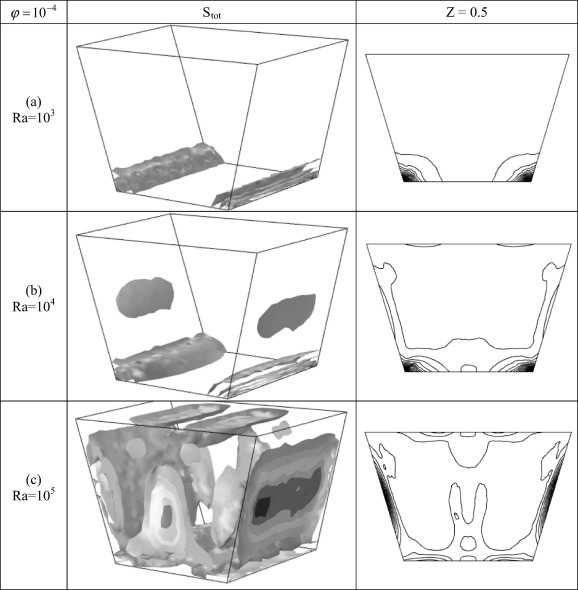Figure 9. The iso-surfaces (left) and isolines (right) of the total entropy generation (Stot) in the cubical trapezoidal cavity [${\textstyle \Phi =0{\mbox{°}}}$] for various values of Rayleigh number [(a) Ra = 103, Ra = 104 and (c) Ra = 105] and φ = 10−4.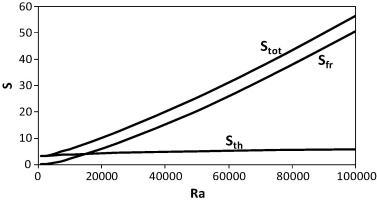Figure 10. The variation of different entropy generation in the cubical trapezoidal cavity [${\textstyle \Phi =0{\mbox{°}}}$] with various values of Rayleigh number.

### 3.5. Effect of inclination angle on the thermal field

Fig. 11 shows the isosurfaces of temperature around the all geometry (upper) and isotherms along the mid-section [Z = 0] (lower) in the cubical trapezoidal cavity at [Ra = 105] and various values of inclination angle [(a) ${\textstyle \Phi =0{\mbox{°}}}$; (b) ${\textstyle \Phi =30{\mbox{°}}}$; (c) ${\textstyle \Phi =60{\mbox{°}}}$; (d) ${\textstyle \Phi =90{\mbox{°}}}$; (e) ${\textstyle \Phi =120{\mbox{°}}}$; (f) ${\textstyle \Phi =150{\mbox{°}}}$ and (g) ${\textstyle \Phi =180{\mbox{°}}}$]. For horizontal cubical trapezoidal cavity [i.e., ${\textstyle \Phi =0{\mbox{°}}}$], the thermal field is governed by the Rayleigh number effect only which is explained previously in Fig. 3. Since, the value of the selected Rayleigh number is high [i.e., Ra = 105], the natural convection effect is strong which causes to make the thermal field represented by isotherms and isosurfaces of temperature are non-uniform. Now, when the cavity inclination angle increases to ${\textstyle \Phi =30{\mbox{°}}}$ and ${\textstyle 60{\mbox{°}}}$ respectively, the effect of natural convection decreases slightly and the disturbance in the isotherms becomes less than the corresponding one which is observed at ${\textstyle \Phi =0{\mbox{°}}}$. Furthermore, the thermal boundary layers adjacent to the hot lower wall become less thicker than that observed at ${\textstyle \Phi =0{\mbox{°}}}$. For vertical cubical trapezoidal cavity [i.e., ${\textstyle \Phi =90{\mbox{°}}}$], the thermal field becomes somewhat uniform indicating the beginning of the conduction mode of the heat transfer inside the cavity. But, when the cavity inclination angle increases to ${\textstyle \Phi =120{\mbox{°}}}$, ${\textstyle 150{\mbox{°}}}$ and ${\textstyle 180{\mbox{°}}}$ respectively, the pattern of isotherms will change completely especially at ${\textstyle \Phi =180{\mbox{°}}}$. The isotherms are uniformly distributed inside the cavity implying conduction as the dominant heat transfer mode.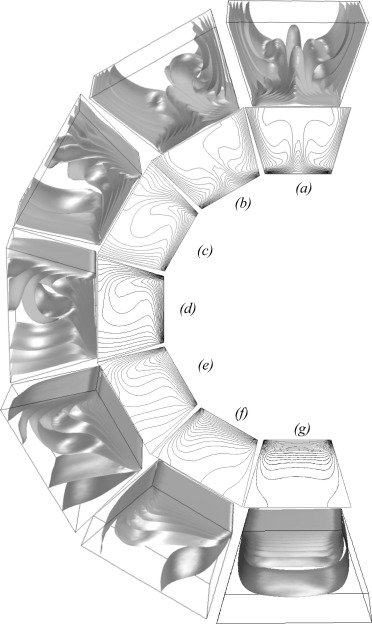Figure 11. The isosurfaces of temperature around the all geometry (upper) and isotherms along the mid-section (lower) in the cubical trapezoidal cavity at [Ra = 105] and various values of inclination angle [(a) ${\textstyle \Phi =0{\mbox{°}}}$; (b) ${\textstyle \Phi =30{\mbox{°}}}$; (c) ${\textstyle \Phi =60{\mbox{°}}}$; (d) ${\textstyle \Phi =90{\mbox{°}}}$; (e) ${\textstyle \Phi =120{\mbox{°}}}$; (f) ${\textstyle \Phi =150{\mbox{°}}}$ and (g) ${\textstyle \Phi =180{\mbox{°}}}$].

### 3.6. Effect of inclination angle on the flow field

Fig. 12 displays the trajectory of particles around the all geometry (upper) and streamlines along the mid-section [Z = 0] (lower) in the cubical trapezoidal cavity at [Ra = 105] and various values of inclination angle [(a) ${\textstyle \Phi =0{\mbox{°}}}$; (b) ${\textstyle \Phi =30{\mbox{°}}}$; (c) ${\textstyle \Phi =60{\mbox{°}}}$; (d) ${\textstyle \Phi =90{\mbox{°}}}$; (e) ${\textstyle \Phi =120{\mbox{°}}}$; (f) ${\textstyle \Phi =150{\mbox{°}}}$ and (g) ${\textstyle \Phi =180{\mbox{°}}}$]. The results demonstrate that the cavity inclination angle has a significant role on the flow pattern. When the inclination angle is ${\textstyle \Phi =0{\mbox{°}}}$ and ${\textstyle 30{\mbox{°}}}$, the flow field inside the cavity can be represented by two symmetrical re-circulating vortices. The effect of buoyancy force in this case is high. But, when the cavity inclination angle increases to ${\textstyle \Phi =60{\mbox{°}}}$ and ${\textstyle 90{\mbox{°}}}$, the flow field inside the cavity is represented by a single large re-circulating vortex. As, the cavity inclination angle jumps to ${\textstyle \Phi =120{\mbox{°}}}$, ${\textstyle 150{\mbox{°}}}$ and ${\textstyle 180{\mbox{°}}}$ respectively, the flow field inside the cavity is characterized again by two unsymmetrical re-circulating vortices. The effect of natural convection in this case begins to decrease gradually as the cavity inclination angle increases.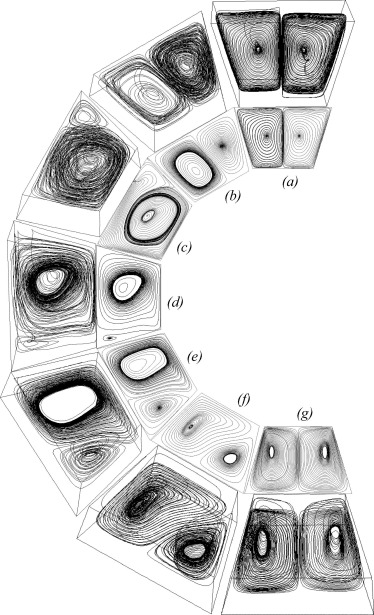Figure 12. The trajectory of particles around the all geometry (upper) and streamlines along the mid-section (lower) in the cubical trapezoidal cavity at [Ra = 105] and various values of inclination angle [(a) ${\textstyle \Phi =0{\mbox{°}}}$; (b) ${\textstyle \Phi =30{\mbox{°}}}$; (c) ${\textstyle \Phi =60{\mbox{°}}}$; (d) ${\textstyle \Phi =90{\mbox{°}}}$; (e) ${\textstyle \Phi =120{\mbox{°}}}$; (f) ${\textstyle \Phi =150{\mbox{°}}}$ and (g) ${\textstyle \Phi =180{\mbox{°}}}$].

### 3.7. Effect of inclination angle on average Nusselt numbers

Fig. 13 illustrates the relationship between the average Nusselt numbers in the cubical trapezoidal cavity and Rayleigh number for various values of inclination angle. It is found that the average Nusselt number reaches its maximum value at ${\textstyle \Phi =30{\mbox{°}}}$, while, the minimum value of the average Nusselt number is reached at ${\textstyle \Phi =180{\mbox{°}}}$. The reason of this behavior is due to the increase of distance between the hot and cold cavity walls when the inclination angle increases to ${\textstyle \Phi =180{\mbox{°}}}$. This increase reduces the temperature gradient and causes to drop the average Nusselt number values. Fig. 14 depicts the relationship between the average Nusselt numbers in the cubical trapezoidal cavity and inclination angle for various values of Rayleigh number. It can be seen that the average Nusselt number is an increasing function of Rayleigh number due to the significant increase in the convection effect as expected. The results show also that when the inclination angle in the range is 0° ⩽ Φ ⩽ 90°, the effect of Rayleigh number is greater than its effect when the inclination angle in the range is 120° ⩽ Φ ⩽ 180°. The reason of this behavior is explained previously.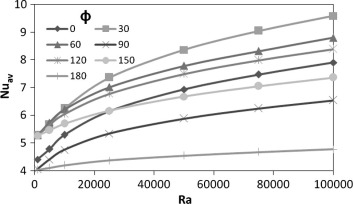Figure 13. The relationship between the average Nusselt numbers in the cubical trapezoidal cavity and Rayleigh number for various values of inclination angle.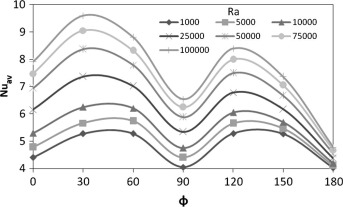Figure 14. The relationship between the average Nusselt numbers in the cubical trapezoidal cavity and inclination angle for various values of Rayleigh number.

### 3.8. Effect of inclination angle on entropy generation

Fig. 15 displays the relationship between the total entropy generation (Stot) in the cubical trapezoidal cavity and inclination angle for various values of Rayleigh number. It can be seen from this figure, that the total entropy generation increases strongly for high Rayleigh number [i.e., Ra = 105] and [${\textstyle \Phi =0{\mbox{°}}}$]. This increase is due to an enhancement in the flow circulation when the Rayleigh number is high. But, as the cavity inclination angle increases, the profiles of the total entropy generation begin to decrease gradually. The result indicated also that the total entropy generation has an approximately linear profile when the Rayleigh number is low. Therefore, one can conclude that the inclination angle effect on the total entropy generation becomes insignificant when the Rayleigh number is low. Fig. 16 illustrates the relationship between the total entropy generation (Stot) in the cubical trapezoidal cavity and Rayleigh number for various values of inclination angle. Similar to the results noticed in Fig. 15, it can be seen that the total entropy generation has a maximum value for horizontal cubical trapezoidal cavity [i.e., ${\textstyle \Phi =0{\mbox{°}}}$], while, it has a minimum value when the inclination angle equals to [${\textstyle \Phi =180{\mbox{°}}}$]. This behavior can be seen for all values of the Rayleigh number. Furthermore, the total entropy generation (Stot) increases as the Rayleigh number increases. But as mentioned previously, this increase is high for horizontal cubical trapezoidal cavity [i.e., ${\textstyle \Phi =0{\mbox{°}}}$], while it decreases as the inclination angle increases.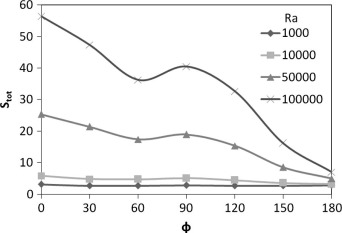Figure 15. The relationship between the total entropy generation (Stot) in the cubical trapezoidal cavity and inclination angle for various values of Rayleigh number.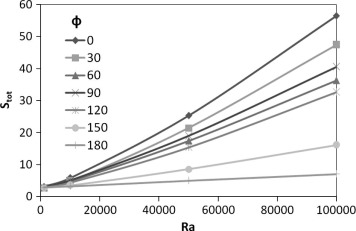Figure 16. The relationship between the total entropy generation (Stot) in the cubical trapezoidal cavity and Rayleigh number for various values of inclination angle.

## 4. Conclusions

The following conclusions can be detected from the results of the present work:

• When the natural convection is weak [i.e., Ra = 103], isotherms are in general smooth, nearly vertical and semi-parallel to the right and left cavity sidewalls and the heat is transferred by conduction.
• When the natural convection is strong [i.e., Ra = 105], the concentrated region of isotherms adjacent to the lower wall becomes more intense and isothermal lines are become more confuse due to strong circulation.
• The difference between the two-dimensional and three-dimensional flow pattern results becomes clear as the Rayleigh number increases.
• When the Rayleigh number increases, the flow circulation increases and some disturbances in the flow patterns are observed especially in 3D results.
• The flow pattern inside the cubical trapezoidal cavity consists of four spiral re-circulating vortices which cover all the span of it.
• The local Nusselt number distribution is almost parallel to the horizontal upper and lower cavity walls.
• When the Rayleigh number increases the average Nusselt number increases.
• The entropy generation contours due to the heat transfer (Sth) increase strongly when the Rayleigh number increases especially adjacent to the heat source location at the lower wall.
• The entropy generation contours due to the fluid friction (Sfr) are concentrated strongly adjacent to the cavity right and left sidewalls.
• The entropy generation due to the heat transfer (Sth) increases slightly as the Rayleigh number increases, while, a strong increase with the Rayleigh number is seen with respect to entropy generation due to friction (Sfr) and the total entropy generation (Stot).
• Different flow patterns can be seen inside the cubical trapezoidal cavity as the inclination angle increases from ${\textstyle \Phi =0{\mbox{°}}}$ to ${\textstyle \Phi =180{\mbox{°}}}$. This gives an important indication that the flow field inside the cavity is significantly affected by the inclination angle.
• When the cavity inclination angle increases, isotherms are uniformly distributed, less compressed inside the cavity and the conduction is the dominant heat transfer mode. Also, the thickness of thermal boundary layer decreases as the cavity inclination angle increases.
• The minimum average Nusselt number inside the cubical trapezoidal cavity corresponds to the highest inclination angle [i.e., ${\textstyle \Phi =180{\mbox{°}}}$], while, the results indicated that the average Nusselt number reaches its maximum value at ${\textstyle \Phi =30{\mbox{°}}}$.
• The inclination angle effect on the total entropy generation becomes insignificant when the Rayleigh number is low.
• The total entropy generation has a maximum value for horizontal cubical trapezoidal cavity [i.e., ${\textstyle \Phi =0{\mbox{°}}}$], while, it has a minimum value when the inclination angle equals to [${\textstyle \Phi =180{\mbox{°}}}$].

### Document informationPublished on 12/04/17

Licence: Other

### Document Score0

Views 27
Recommendations 0